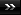View Single Post01-21-2010, 07:59 AM   #708
abraxasinas
_

Join Date: Dec 2009
Location: Queanbeyan/Canberra; NSW, Australia
Posts: 635Re: Thuban Q&A: (warning longer than normal posts here)

Quote:
 Originally Posted by PhthaThanks Sirebard that is what I figured about energy. So we get all energy from this single supersource, and depending on the instrument we use to 'draw it' so to speak, is how it manifests itself in many different material forms, which are really just octaves(?) of each other, with of course all the 'tones' or grades in between. And through the harmonics that these instrument creat by just tapping into the energy, is how we access or are aware of the 'higher' or less distorted energies? Not so much octaves phtha; but a musician would be able to describe this better than me and know how to relate the musical harmonies and octaves to this superenergy (which you have intuited appropriately here relative the Thuban sciences). I am musically illiterate. The harmonics are more like the 'Harmony of the Spheres' after Pythagoras and Kepler. In the physics it becomes the famous Schroedinger Wave Equation, say as the harmonic bouncing of a 'particle' (as the universe) between two nodes as a Standing Wave Harmonic. Then the 'harmonics' obey the laws of quantum mechanics in the wavelength partitionings and such. And yes, the MUSIC, just as the MATHEMATICS form the UNIVERSAL LANGUAGE connecting all dimensions and densities. The FEELING Modes of the SPIRIT then KNOW instinctively or intuitively or telepathically of how to INTERPRET the 'harmonics' THROUGH the FEELINGS int activities, such as Dancing. Some simple mathematical harmonics are: The Beauty of Mathematics 1 x 8 + 1 = 9 12 x 8 + 2 = 98 123 x 8 + 3 = 987 1234 x 8 + 4 = 9876 12345 x 8 + 5 = 98765 123456 x 8 + 6 = 987654 1234567 x 8 + 7 = 9876543 12345678 x 8 + 8 = 98765432 123456789 x 8 + 9 = 987654321 1 x 9 + 2 = 11 12 x 9 + 3 = 111 123 x 9 + 4 = 1111 1234 x 9 + 5 = 11111 12345 x 9 + 6 = 111111 123456 x 9 + 7 = 1111111 1234567 x 9 + 8 = 11111111 12345678 x 9 + 9 = 111111111 123456789 x 9 +10= 1111111111 9 x 9 + 7 = 88 98 x 9 + 6 = 888 987 x 9 + 5 = 8888 9876 x 9 + 4 = 88888 98765 x 9 + 3 = 888888 987654 x 9 + 2 = 8888888 9876543 x 9 + 1 = 88888888 98765432 x 9 + 0 = 888888888 Brilliant, isn't it? And look at this symmetry: 1 x 1 = 1 11 x 11 = 121 111 x 111 = 12321 1111 x 1111 = 1234321 11111 x 11111 = 123454321 111111 x 111111 = 12345654321 1111111 x 1111111 = 1234567654321 11111111 x 11111111 = 123456787654321 111111111 x 111111111 = 12345678987654321 Mind Boggling... Now, take a look at this... 101% >From a strictly mathematical viewpoint: What Equals 100%? What does it mean to give MORE than 100%? Ever wonder about those people who say they are giving more than 100%? We have all been in situations where someone wants you to GIVE OVER 100%... How about ACHIEVING 101%? What equals 100% in life? Here's a little mathematical formula that might help answer these questions: If: A B C D E F G H I J K L M N O P Q R S T U V W X Y Z Is represented as: 1 2 3 4 5 6 7 8 9 10 11 12 13 14 15 16 17 18 19 20 21 22 23 24 25 26. Then: H-A-R-D-W-O- R- K 8+1+18+4+23+15+18+11 = 98% And: K-N-O-W-L-E- D-G-E 11+14+15+23+12+5+4+7+ 5 = 96% But: A-T-T-I-T-U- D-E 1+20+20+9+20+ 21+4+5 = 100% THEN, look how far the love of God will take you: L-O-V-E-O-F- G-O-D 12+15+22+5+15+ 6+7+15+4 = 101% Therefore, one can conclude with mathematical certainty that: While Hard Work and Knowledge will get you close, and Attitude will get you there, It's the Love of God that will put you over the top! SAEPE EXPERTUS, SEMPER FIDELIS, FRATRES AETERNI (Often Tested, Always Faithful, Brothers Forever) __._,_.___ And I have read through those, still lots of contemplation before I get a realization yet thought.AA